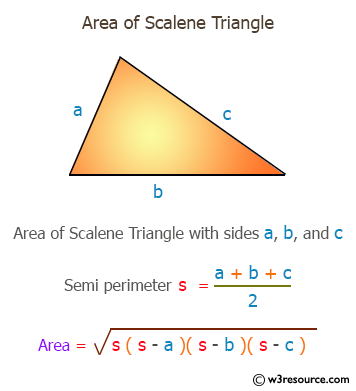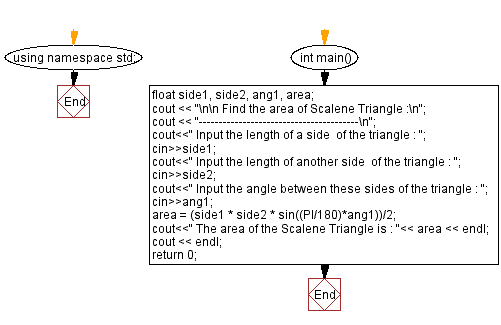﻿ C++ Exercises: Find the area of Scalene Triangle - w3resource

# C++ Exercises: Find the area of Scalene Triangle

## C++ Basic: Exercise-28 with Solution

Write a C++ program to find the area of the Scalene Triangle.

Pictorial Presentation:Sample Solution:

C++ Code :

``````#include <iostream>
#include <math.h>
#define PI 3.14159
using namespace std;

int main()
{
float side1, side2, ang1, area;
cout << "\n\n Find the area of Scalene Triangle :\n";
cout << "----------------------------------------\n";
cout<<" Input the length of a side  of the triangle : ";
cin>>side1;
cout<<" Input the length of another side  of the triangle : ";
cin>>side2;
cout<<" Input the angle between these sides of the triangle : ";
cin>>ang1;
area = (side1 * side2 * sin((PI/180)*ang1))/2;
cout<<" The area of the Scalene Triangle is : "<< area << endl;
cout << endl;
return 0;
}
``````

Sample Output:

``` Find the area of Scalene Triangle :
----------------------------------------
Input the length of a side  of the triangle : 5
Input the length of another side  of the triangle : 6
Input the angle between these sides of the triangle : 6
The area of the Scalene Triangle is : 1.56793
```

Flowchart:C++ Code Editor:

What is the difficulty level of this exercise?

﻿

## C++ Programming: Tips of the Day

Why is there no std::stou?

The most pat answer would be that the C library has no corresponding "strtou", and the C++11 string functions are all just thinly veiled wrappers around the C library functions: The std::sto* functions mirror strto*, and the std::to_string functions use sprintf.

Ref: https://bit.ly/3wtz2qA

We are closing our Disqus commenting system for some maintenanace issues. You may write to us at reach[at]yahoo[dot]com or visit us at Facebook